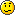# CCIE Level question

Member Posts: 447 ■■■□□□□□□□
Calculating flows for WFQ.

Flow 1 - 500 byte packets with IP precedence 3.
Flow 2 - 250 byte packets with IP precedence 3.
Flow 3 - 250 byte packets with IP precedence 1.

For every 1 packet of flow 1 that is serviced, how many packets of flow 2 and flow 3 will be serviced?

• I have no clue, but my guess is:

Uhhh, WFQ=WTF!!!1/2 packet for flow 2,and 1/2 for flow 2...????? Sorry, I have never touched a Cisco book, I just like trivia...
i remain, he who remains to be....
• Member Posts: 726 ■■■■□□□□□□
mama!!!CCNP Security - DONE!
CCNP R&S - In Progress...
CCIE Security - Future...
• tunerX wrote:
Calculating flows for WFQ.

Flow 1 - 500 byte packets with IP precedence 3.
Flow 2 - 250 byte packets with IP precedence 3.
Flow 3 - 250 byte packets with IP precedence 1.

For every 1 packet of flow 1 that is serviced, how many packets of flow 2 and flow 3 will be serviced?

I'm going to assume the weight being used is post IOS 12.0(5)T.

Let's see, the weight for flow 1 would be....

32384 / 2000 = 16.192

Flow 2 would be

32384 / 1000 = 32.384

Flow 3 would be

32384 / 500 = 64.768

So for every one packet that flow 1 gets through, flow 2 will get two through and flow 3 will get 4 through.

At least, I thinkQoS isn't something I'm delving into in depth at present.
= Marcus Drakonblayde
================
CCNP-O-Meter:
=[0%]==[25%]==[50%]==[75%]==[100%]
==[X]===[X]====[ ]=====[ ]====[ ]==
=CCNA==BSCI==BCMSN==BCRAN==CIT=
• I'm going to assume the weight being used is post IOS 12.0(5)T.

Let's see, the weight for flow 1 would be....

32384 / 2000 = 16.192

Flow 2 would be

32384 / 1000 = 32.384

Flow 3 would be

32384 / 500 = 64.768

So for every one packet that flow 1 gets through, flow 2 will get two through and flow 3 will get 4 through.

At least, I think icon_smile.gif QoS isn't something I'm delving into in depth at present.

Wow! Man...... I thought addition was hardWIP Vacation ;-)

Porsche..... there is no substitute!
• hehe, I'm not sure if I'm right, I remember reading over Weighted Fair Queueing in one of my books, and I had to look up the weight for the post 12.0(5)T IOS (pre it's 4096), but after that, it's a fairly simple formula...

Weight = 32384 ( or 4096) / (IP Precedence + 1) * PacketSize

After that, just do the math and whatever comes out with the higher weight has more priority
= Marcus Drakonblayde
================
CCNP-O-Meter:
=[0%]==[25%]==[50%]==[75%]==[100%]
==[X]===[X]====[ ]=====[ ]====[ ]==
=CCNA==BSCI==BCMSN==BCRAN==CIT=
• Member Posts: 447 ■■■□□□□□□□
You got the formula right but the weights backwards. With WFQ lower weights are better.

flow 1: 32384/(3+1)=8096
flow 2: 32384/(3+1)=8096
flow 3: 32384/(1+1)=16192

If we assume that all flows started at the same time. The initial value of the SN (sequence numbers) for each flow will be zero. To make things easier, I will drop all of the numbers on the right.

flow 1: SN1=(8096*500)=4048000
flow 2: SN1=(8096*250)=2024000
flow 3: SN1=(16192*250)=4048000

all following serial numbers are calculated by adding the previous serial number to the product of weight and length.

SN = (previous SN + (weight*length))

On average for every 1 packet that flow 1 sends, flow 2 will have sent 2 and flow 3 will send 1.

Queue
F1: SN3~12, SN2~8, SN1~4
F2: SN3~6, SN2~4, SN1~2
F3: SN3~12, SN2~8, SN1~4
Queue
• tunerX wrote:
You got the formula right but the weights backwards. With WFQ lower weights are better.

DOH! Well, I'll never forget that little tidbit againHrm, my math was also wrong. I guess the proper way for the formula then would be Weight = (32384 / (IP Precedence + 1)) * PacketSize.
= Marcus Drakonblayde
================
CCNP-O-Meter:
=[0%]==[25%]==[50%]==[75%]==[100%]
==[X]===[X]====[ ]=====[ ]====[ ]==
=CCNA==BSCI==BCMSN==BCRAN==CIT=